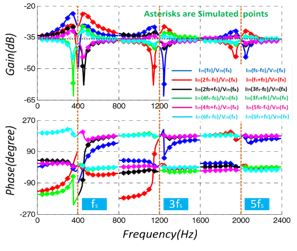LIBRARY

# Modeling and Analysis for Input Characteristics of Line-Frequency Rectifiers

Year: 2017 | Author: Xiaolong Yue | Paper: D2.12Fig. 1. Simulation for the proposed model
Impedance is very important for power electronic systems because of the close relationship between impedance and system stability. The line-frequency rectifiers are commonly used ac-dc interfaces in electrical power systems. For the line-frequency rectifier with a sinusoidal voltage perturbation excitation at the input terminal, input current contains not only perturbation frequency, but also multiple additional frequency components. Therefore, input characteristics of line-frequency rectifiers are actually single input multiple output (SIMO) in frequency domain. However, input current perturbation is traditionally simplified into a sinusoidal signal at perturbation frequency by ignoring other additional frequency components. The obtained impedance models mainly focus on frequency regions below line frequency in order to describe the SIMO input characteristics of line-frequency rectifiers and to develop an impedance model that could be applied beyond switching frequency.

This paper proposes a new model by using the harmonic balance method. Detailed analysis indicates that input admittance of the line frequency rectifier in high frequency regions acts like a resistor rather than an inductor. The simulations validate the accuracy and effectiveness of the proposed model.

For nonlinear power electronic converters, the definition of impedance makes classical control theory easy to use . This solves many problems and brings lots of convenience, but the simplification of real input characteristics also has some limitations. It has been reported that the output impedance model fails to analyze the multi-phase voltage regulator's characteristics when there is a load transient whose frequency is beyond switching frequency and power electronic based systems' interactions in switching frequency range.

Fig.1 shows the simulated input current and voltage waveforms with perturbations. Simulation results coincide with theoretical values, which validates the accuracy of the proposed model.

The describing function (input admittance) iin(fx)/vin fx can be obtaines as shown in Fig. 2. The figure shows that the highest gains appear around line frequency and the distance between peak points and line frequency is resonant frequency of output low pass filter.Fig. 2. Input admittance of line-frequency rectifier